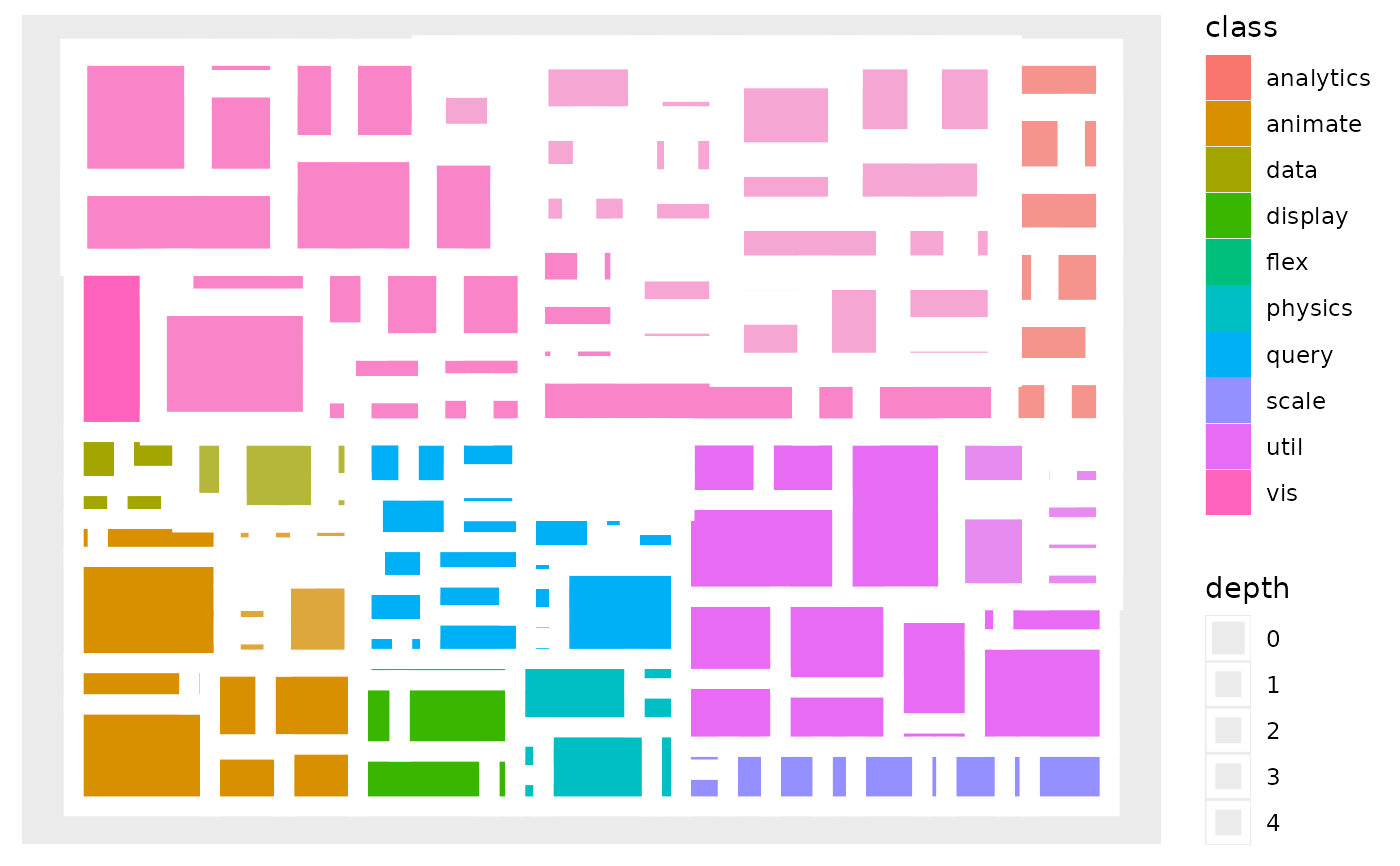A treemap is a space filling layout that recursively divides a rectangle to the children of the node. Often only the leaf nodes are drawn as nodes higher up in the hierarchy would obscure what is below. geom_treemap is a shorthand for geom_node_treemap as node is implicit in the case of treemap drawing

geom_node_tile(
mapping = NULL,
data = NULL,
position = "identity",
show.legend = NA,
...
)

## Arguments

mapping

Set of aesthetic mappings created by ggplot2::aes() or ggplot2::aes_(). By default x, y, width and height are mapped to x, y, width and height in the node data.

data

The data to be displayed in this layer. There are three options:

If NULL, the default, the data is inherited from the plot data as specified in the call to ggplot().

A data.frame, or other object, will override the plot data. All objects will be fortified to produce a data frame. See fortify() for which variables will be created.

A function will be called with a single argument, the plot data. The return value must be a data.frame, and will be used as the layer data. A function can be created from a formula (e.g. ~ head(.x, 10)).

position

Position adjustment, either as a string, or the result of a call to a position adjustment function.

show.legend

logical. Should this layer be included in the legends? NA, the default, includes if any aesthetics are mapped. FALSE never includes, and TRUE always includes. It can also be a named logical vector to finely select the aesthetics to display.

...

Other arguments passed on to layer(). These are often aesthetics, used to set an aesthetic to a fixed value, like colour = "red" or size = 3. They may also be parameters to the paired geom/stat.

## Aesthetics

geom_treemap understand the following aesthetics. Bold aesthetics are automatically set, but can be overridden.

• x

• y

• width

• height

• alpha

• colour

• fill

• size

• stroke

• filter

Other geom_node_*: geom_node_arc_bar(), geom_node_circle(), geom_node_point(), geom_node_range(), geom_node_text(), geom_node_voronoi()

## Author

Thomas Lin Pedersen

## Examples

# Create a graph of the flare class system
library(tidygraph)
flareGraph <- tbl_graph(flare$vertices, flare$edges) %>%
mutate(
class = map_bfs_chr(node_is_root(), .f = function(node, dist, path, ...) {
if (dist <= 1) {
return(shortName[node])
}
path\$result[[nrow(path)]]
})
)
#> Warning: Argument neimode' is deprecated; use mode' instead

ggraph(flareGraph, 'treemap', weight = size) +
geom_node_tile(aes(fill = class, filter = leaf, alpha = depth), colour = NA) +
geom_node_tile(aes(size = depth), colour = 'white') +
scale_alpha(range = c(1, 0.5), guide = 'none') +
scale_size(range = c(4, 0.2), guide = 'none')